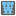• # most wanted letter

## Question related to missionThe Most Wanted Letter

def checkio(text): n = 0 s = text.lower() for i in s: if i.isalpha() and n < s.count(i): n = s.count(i) r = "z" for i in s: if i.isalpha() and s.count(i) == n and i <= r: r = i return r

Can someone explain to me why s.count(i) == n returns only the most frequent letter